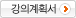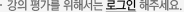### 주메뉴

### 전자물성

• 경북대학교
• 김학린
• 주제분류
공학 >전기ㆍ전자 >전자공학
• 강의학기
2018년 2학기
• 조회수
1,749
•
강의계획서The goal of this graduate-level class is to understand more advanced physics and electrical properties of semiconductors.

- Introducing the classification of materials and basic properties of semiconductors

- Discussing basics of quantum mechanics that are required to understand semiconductor properties

- Discussing energy band theory and equilibrium carrier concentration in semiconductors

- Discussing the charge transports in semiconductors

#### 차시별 강의1Class overview and introduction to semiconductors Class overview and introduction to semiconductors2The crystal structure of semiconductors The crystal structure of semiconductors3Basics of quantum mechanics for semiconductors (1) Basics of quantum mechanics for semiconductors (1)4Basics of quantum mechanics for semiconductors (2) Basics of quantum mechanics for semiconductors (2)5Equlibrium carrier statics (1) - Density of states Equlibrium carrier statics (1) - Density of states6Equilibrium carrier statics (2) - Equilibrium carrier concentration Equlibrium carrier statics (2) - Equilibrium carrier concentration7Equlibrium carrier statics (3) : Concentration and Fermi level calculations, Determination of Fermi level Equlibrium carrier statics (3) - Concentration and Fermi level calculations - Determination of Fermi level8Recombination-generation processes Recombination-generation processes9Carrier transport (1) - Drift Carrier transport (1) - Drift10Carrier transport (2) - Diffusion Carrier transport (2) - Diffusion11Carrier transport (3) - Continuity equations and diffusion equations Carrier transport (3) - Continuity equations and diffusion equations12Carrier transport (3) - Continuity equations and diffusion equations 2 Carrier transport (3) - Continuity equations and diffusion equations#### 연관 자료#### 사용자 의견#### 이용방법

• 문서 자료 이용시 필요한 프로그램 [바로가기]

※ 강의별로 교수님의 사정에 따라 전체 차시 중 일부 차시만 공개되는 경우가 있으니 양해 부탁드립니다.